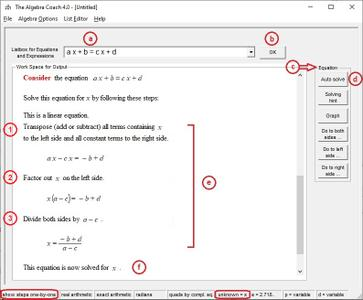# Algebra Coach 4.0Algebra Coach 4.0 | 4.9 Mb
The Algebra Coach is an app that can help you learn algebra. It explains algebra step-by-step exactly the way the teacher does. It is sophisticated but still easy to understand and use.

The Algebra Help e-book also contains exercise problems that you can drag-and-drop into the Algebra Coach.

The Algebra Coach can also teach you how to manipulate an expression - simplify, factor, expand, complete the square, and so on.

The Algebra Coach can also teach you how to prove a trigonometric identity. Click here to see details on that.

The Algebra Coach can solve every type of equation that you will encounter in high school or college algebra. Here is a (particularly nasty looking) rational equation. This equation requires about 20 steps to arrive at the solution and in step-by-step mode you would see all 20 of them. In result-only mode you see the result only. In exact mode the solution is shown as fractions. In floating point mode it would be shown as decimal numbers.

What you have seen is just the tip of the iceberg. The Algebra Coach can do much, much more.

``https://mathonweb.com/``
•••